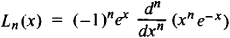# Laguerre Polynomials

(redirected from Laguerre functions)

## Laguerre polynomials

[lə′ger ‚päl·ə′nō·mē·əlz]
(mathematics)
A sequence of orthogonal polynomials which solve Laguerre's differential equation for positive integral values of the parameter.

## Laguerre Polynomials

(named after the French mathematician E. Laguerre, 1834–86), a special system of polynomials of successively increasing degree. For n = 0, 1, 2, … the Laguerre polynomials Ln(x) can be defined by the formulaThe first few Laguerre polynomials are

L0(x) = 1, L1(x) = x – 1, l2(x) = x2 – 4x + 2

L3(x) = x3 – 9x2 + 18x – 6

The Laguerre polynomials are orthogonal on the half-line x ≥ 0 with respect to the weight function e–x and are solutions of the differential equation

xyn + (1 – x)y + ny = 0

The Laguerre polynomials are recursively defined by the formula

Ln+1(x) = (x – 2n – 1)Ln(x) – n2Ln–1(x)

### REFERENCE

Lebedev, N. N. Spetsial’nye funktsii i ikh prilozheniia, 2nd ed. Moscow-Leningrad, 1963.
Mentioned in ?
References in periodicals archive ?
Parameters that appear in the solution but not in the partial-differential equation take discrete values, imposed by boundary conditions, as follows: m is called the equatorial, or magnetic, quantum number that assumes only integer values and that arises in the solution of the angular equation to define [PHI]([phi]), as in spherical polar coordinates; the first arguments of the associated Laguerre functions, m and n2, like radial quantum number k among the three quantum numbers pertaining to spherical polar coordinates, must be non-negative integers so that for bound states of the hydrogen atom the Laguerre functions in U(u) and V(v) terminate at finite powers of variable u or v, and remain finite for u or v taking large values, respectively.
Just as a Laguerre polynomial in R(r) in spherical polar coordinates contains a sum of quantum numbers that occurs also in the exponent of the temporal factor, so both U(u) and V(v) contain, in the third arguments of their Laguerre functions, a sum n1 + n2 + [absolute value of m] + 1; that sum that must take values of a positive integer likewise occurs in the temporal factor, omitted above; we associate that sum with n, an integer quantum number for energy that was originally defined from experiment.
The method of approximation analyzed in this paper is the Laguerre Impulse Response Approximation (LIRA) which is based on approximation of noninteger order system impulse response with Laguerre functions.
2]([0, [infinity]], R), we will consider orthonormal Laguerre functions denoted by [e.
MPC implementation based on Laguerre functions also called Laguerre optimal model predictive control (LOMPC) has been discussed in [11,12] enables the capture of near optimal control trajectory with less number of parameters as compared with conventional MPC algorithms.
The novel contribution is the comparison of the performance and online computation time of variants of MPC including GPC, nominally stable (closed-loop paradigm, dual- mode) MPC and Laguerre function based MPC and a PID controller in simulation for the trajectory control of a Quadrotor helicopter
The Rao-Wilton-Glisson (RWG)  functions are used as the spatial expansion and testing functions, and the weighted Laguerre functions are used as the temporal expansion and testing functions.
M) separately to the Equation (47), where M is the maximum order of the Laguerre functions to be evaluated from the time-bandwidth product of the waveform.
In this paper, we propose an efficient and accurate method to apply FDTD for transient wave propagation in a general dispersive media using the MOD scheme with the associate Laguerre functions.
The most important special functions are known as: Bessel functions, Hermite functions, Legendre functions, Laguerre functions, Chebyshev functions etc.
We remark that we have studied theWeyl-Bessel transforms in , and theWeyl transforms associated with Laguerre functions in .
After conversion from Whittaker functions in Maple's direct solution of the ordinarydifferential equations for U(u) and V(v) because Schroedinger's equation in these coordinates is a special case of Whittaker's differential equation, through Kummer functions to preserve the phase characteristics, to associated Laguerre functions  that both U(u) and V(v) contain, and setting the first arguments of these Laguerre functions to non-negative integers ni and n2, respectively, the algebraic solutions for the coordinates of length type become

Site: Follow: Share:
Open / Close